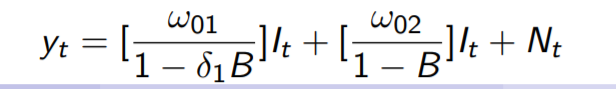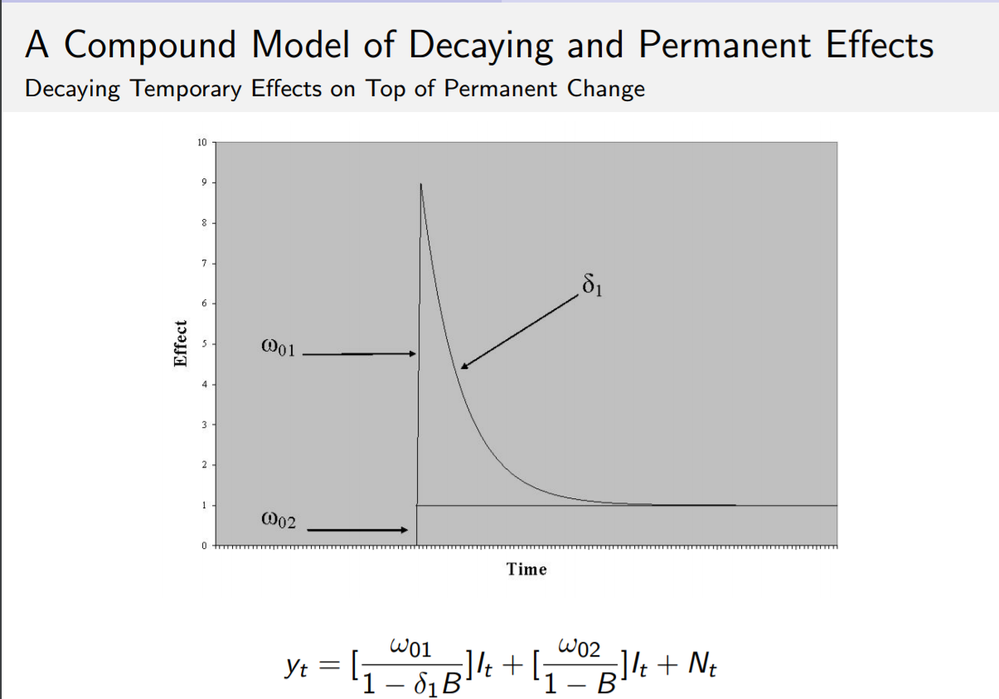## ARIMA transfer function compound effect

Hello, I would l would like to know how one could specify the following compounded transfer function. This gives a compounded effect of abrupt spike and decay and settles in a new mean.This can be visualized as follows. I can easily code the first part of the equation, the question is how to compound both with same intervention variable. Thanks in advance## Re: ARIMA transfer function compound effect

This is a good question.  If your second transfer function spec did not have a denominator polynomial with unit root, you could have easily specified this model by including an extra copy of your I variable, say copy_I, in your input data set.  As it happens, the denominator polynomials in ARIMA cannot be "unstable".  One way to get around this and specify a model that is reasonably close to your model is as follows (this still involves making a copy of the I variable):

Note that after multiplying your model equation by (1-B), one gets the following:

(1-B) y_t = (omega_01/(1-delta B)) (1-B) X_t + omega_02 X_t + (1-B) N_t.

I have renamed your I variable as X.  For simplicity, let us assume that your noise process N_t is a simple white noise.  Then (1-B) N_t is an MA(1) process with MA parameter equal to 1 (which is noninvertible).  In ARIMA you can specify a model close to this rearranged model where the noise is invertible MA(1).  You could do this as follows:

Suppose your input data set is TEST.

Step1.  Create a copy of variable X:

data test;

set test;

copy_X = X;

run;

Step 2: Specify the model:

proc arima data=test;
i var=y(1) crosscorr=(X copy_X(1)) noprint;
e q=1 input=(/(1) copy_X X) noint method=ml;
quit;

This will give you a specification reasonably close to what you want.  If in fact, the true noise process is non-invertible MA(1), your estimated MA parameter will be close to it.

Hope this works OK for you.

Discussion stats